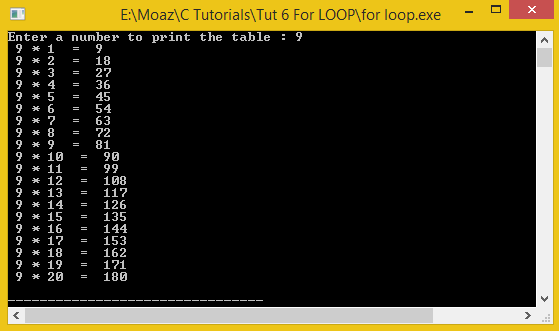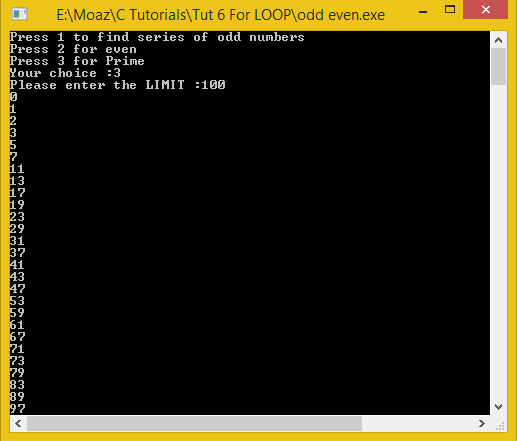# Learning LOOPS in C

In this part you will learn: 1. What is a loop 2. For LOOP 3. More on conditional statements 4. C syntax 5. Showing output In this tutorial I will teach you how to make a calculator using two different ways, firstly using the Conditional statement method and then using switch statement. What is a LOOP ? A loop is simply a statement which can execute a set of defined statements repetitively. You can write any valid C statement within a loop and you can also set how many times to execute that certain statement. There are three parts in a loop 1. Initialization ( loop starting form number mostly 1 or 0 ) 2. Condition ( to stop the loop at certain point ) 3. Increment ( adding a certain number in variable to reach the stopping condition ) Three types of loops in C are 1. For loop 2. While loop 3. Do While loop Today we will discuss for loop. Printing a table of any number using FOR LOOP: Basic Step: Open Dev C++ then File > new > source file and start writing the code below.
`#include<stdio.h>#include<conio.h>`
These are the header files that we need for coding. The main header is stdio.h which provides us with most of the functions for coding in C and the second header files is just for using a function which can pause the screen until the user hits any key.
`int main(){	int number = 1;int count  = 1; //to start the number multiplying by 1	int ans = 0;`
First of all we will declare 3 integers named as ‘num1’,’num2’ and ’ans’, here we have declared 3 integers because in a table we will need two integer type variables on which a specific operation will be performed and the result after the operation will be stored in the third integer type variable. In this program we will only use these variables to take input and storing result as well. In the end the multiplication table will be displayed on the console.
`	printf("Enter a number to print the table : ");	scanf("%d",&number);`
Now we are taking inputs from the user, The user will enter the number and then we will run a loop to find the table of that number.
`for(count =1; count<=20;count++){		ans = number * count;	printf(" %d * %d  = % d\n",number,count,ans); }//end for}//end main`
In the for loop notice that there are two semi colon’s which divides the bracket into three parts. The condition before first semicolon is the initialization. The condition before second semi colon is condition and the condition after semi colon is the increment. We will simply multiply the number (given by user) with the for loop variable count which will start from 1 and go till 20. Incrementing every time the for loop runs. Then we end the program. Execute > compile then Execute > run OutputPrinting EVEN ODD AND PRIME NUMBER SERIES using loops File > new source file
`#include<stdio.h>#include<conio.h> int main(){		int number = 1;int count  = 1; //to start the number multiplying by 1int prime; //loop countersint choice;	int notPrime = 0; // 1 for true and 0 for falseint ans = 0;`
Simply writing the headers and declaring the variables number for defining the limit to print the series. Count for loop , prime variable for loop , choice for switching , notPrime variable is a flag (1 represents true and 0 will represent false ), ans variable to store the answer.
`printf("Press 1 to find series of odd numbers\nPress 2 for even \nPress 3 for Prime\nYour choice :");scanf("%d",&choice);printf("Please enter the LIMIT :");scanf("%d",&number);`
Here we are simply taking the user choice for ODD ,EVEN OR PRIME number series and then we take the input of the limit to which he wants the series to be printed
`switch(choice){	case 1:			// ODD NUMBRERS 		for(count =1; count<=number;count++){			if(count % 2 != 0)			printf("%d\n",count);		}//end for		break;`
In the switch statement we write 3 cases for switching between odd even and prime. The first case is for odd in this case we will run a for loop from 1 to the number (given by user) and check if it has remainder is not equal to 0 when it is divided by 2. if the remainder is not equal 0 when divided by 2 then it is an odd number so we simply print it and move on to the next number of the loop.(REMEMBER % is called modulus operator used to find remainder of a number ).
`case 2:			//	 EVEN NUMBRERS 		for(count =1; count<=number;count++){			if(count % 2 == 0)			printf("%d\n",count);		}//end for		break;`
In this case we will find the even numbers we simply run a loop from 1 to number (given by user) and check if the loop variable if it’s divisible by 2 if yes then it is an even number so we simple print it.
`case 3:		// prime numbers	for(count = 0 ; count<number; count++){		notPrime = 0;		for( prime = 2; prime < count; prime++){			if (count%prime == 0)			   notPrime = 1;			}			if(notPrime == 0)			   printf("%d\n",count);			}//end for		break;default:	printf("Invalid choice");		} 	getch();	return 0;}//end main`
In the third case we are finding the prime number series for that we need two nested for loops (loop within a loop ). One loop for printing the numbers and other one for checking whether the number is prime or not. First we will set the prime variable to 0. Then we will run a 2nd loop and then check the modulus of the every variable of first loop by the complete second loop. Then after checking we will simply check if the number is prime then print otherwise end. Execute > compile then Execute > run Output: